#### The fundamental group

Now an interesting group will be constructed from the space of paths and the equivalence relation obtained by homotopy. The fundamental group,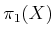(or first homotopy group), is associated with any topological space,. Let a (continuous) path for which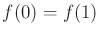be called a loop. Let some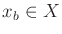be designated as a base point. For some arbitrary but fixed base point,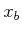, consider the set of all loops such that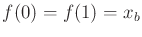. This can be made into a group by defining the following binary operation. Let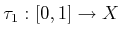andbe two loop paths with the same base point. Their product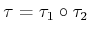is defined as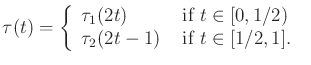(4.9)

This results in a continuous loop path because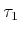terminates at, and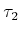begins at. In a sense, the two paths are concatenated end-to-end.

Suppose now that the equivalence relation induced by homotopy is applied to the set of all loop paths through a fixed point,. It will no longer be important which particular path was chosen from a class; any representative may be used. The equivalence relation also applies when the set of loops is interpreted as a group. The group operation actually occurs over the set of equivalences of paths.

Consider what happens when two paths from different equivalence classes are concatenated using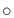. Is the resulting path homotopic to either of the first two? Is the resulting path homotopic if the original two are from the same homotopy class? The answers in general are no and no, respectively. The fundamental group describes how the equivalence classes of paths are related and characterizes the connectivity of. Since fundamental groups are based on paths, there is a nice connection to motion planning.

Example 4..8 (A Simply Connected Space)   Suppose that a topological spaceis simply connected. In this case, all loop paths from a base pointare homotopic, resulting in one equivalence class. The result is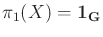, which is the group that consists of only the identity element.Example 4..9 (The Fundamental Group of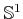)   Suppose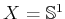. In this case, there is an equivalence class of paths for each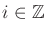, the set of integers. If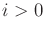, then it means that the path windstimes aroundin the counterclockwise direction and then returns to. If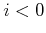, then the path winds aroundtimes in the clockwise direction. If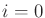, then the path is equivalent to one that remains at. The fundamental group is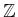, with respect to the operation of addition. Iftravels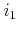times counterclockwise, andtravelstimes counterclockwise, thenbelongs to the class of loops that travel around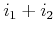times counterclockwise. Consider additive inverses. If a path travels seven times around, and it is combined with a path that travels seven times in the opposite direction, the result is homotopic to a path that remains at. Thus,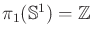.Example 4..10 (The Fundamental Group of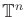)   For the torus,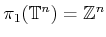, in which theth component of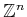corresponds to the number of times a loop path wraps around theth component of. This makes intuitive sense becauseis just the Cartesian product ofcircles. The fundamental groupis obtained by starting with a simply connected subset of the plane and drilling outdisjoint, bounded holes. This situation arises frequently when a mobile robot must avoid collision withdisjoint obstacles in the plane.By now it seems that the fundamental group simply keeps track of how many times a path travels around holes. This next example yields some very bizarre behavior that helps to illustrate some of the interesting structure that arises in algebraic topology.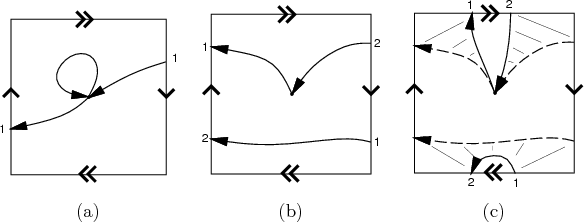Example 4..11 (The Fundamental Group of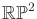)   Suppose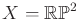, the projective plane. In this case, there are only two equivalence classes on the space of loop paths. All paths that wrap around'' an even number of times are homotopic. Likewise, all paths that wrap around an odd number of times are homotopic. This strange behavior is illustrated in Figure 4.7. The resulting fundamental group therefore has only two elements: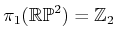, the cyclic group of order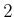, which corresponds to addition mod. This makes intuitive sense because the group keeps track of whether a sum of integers is odd or even, which in this application corresponds to the total number of traversals over the square representation of. The fundamental group is the same for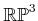, which arises in Section 4.2.2 because it is homeomorphic to the set of 3D rotations. Thus, there are surprisingly only two path classes for the set of 3D rotations.Unfortunately, two topological spaces may have the same fundamental group even if the spaces are not homeomorphic. For example,is the fundamental group of, the cylinder,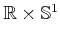, and the Möbius band. In the last case, the fundamental group does not indicate that there is a twist'' in the space. Another problem is that spaces with interesting connectivity may be declared as simply connected. The fundamental group of the sphere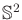is just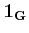, the same as for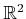. Try envisioning loop paths on the sphere; it can be seen that they all fall into one equivalence class. Hence,is simply connected. The fundamental group also neglects bubbles in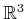because the homotopy can warp paths around them. Some of these troubles can be fixed by defining second-order homotopy groups. For example, a continuous function,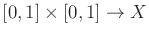, of two variables can be used instead of a path. The resulting homotopy generates a kind of sheet or surface that can be warped through the space, to yield a homotopy group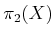that wraps around bubbles in. This idea can be extended beyond two dimensions to detect many different kinds of holes in higher dimensional spaces. This leads to the higher order homotopy groups. A stronger concept than simply connected for a space is that its homotopy groups of all orders are equal to the identity group. This prevents all kinds of holes from occurring and implies that a space,, is contractible, which means a kind of homotopy can be constructed that shrinksto a point . In the plane, the notions of contractible and simply connected are equivalent; however, in higher dimensional spaces, such as those arising in motion planning, the term contractible should be used to indicate that the space has no interior obstacles (holes).

An alternative to basing groups on homotopy is to derive them using homology, which is based on the structure of cell complexes instead of homotopy mappings. This subject is much more complicated to present, but it is more powerful for proving theorems in topology. See the literature overview at the end of the chapter for suggested further reading on algebraic topology.

Steven M LaValle 2020-08-14